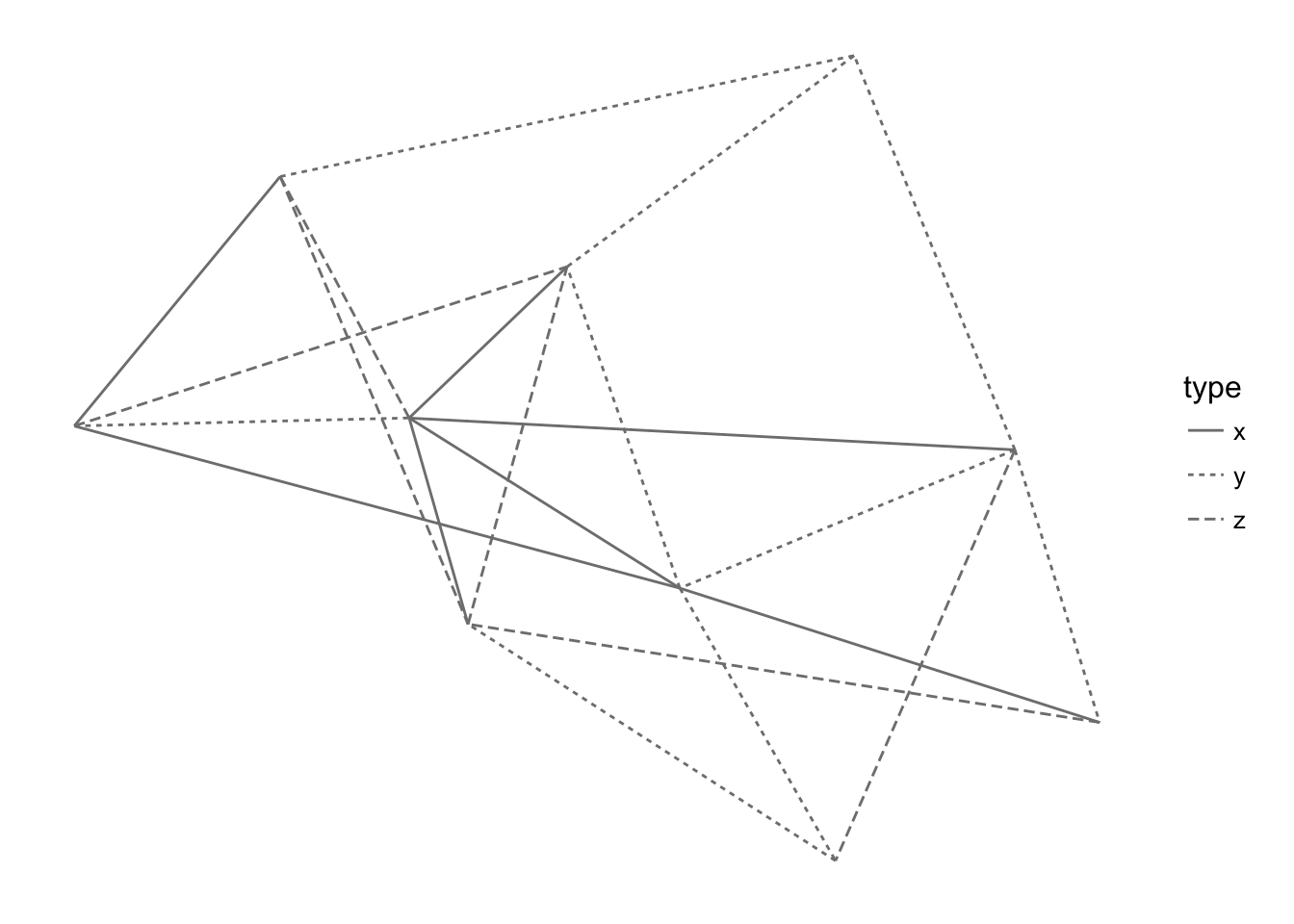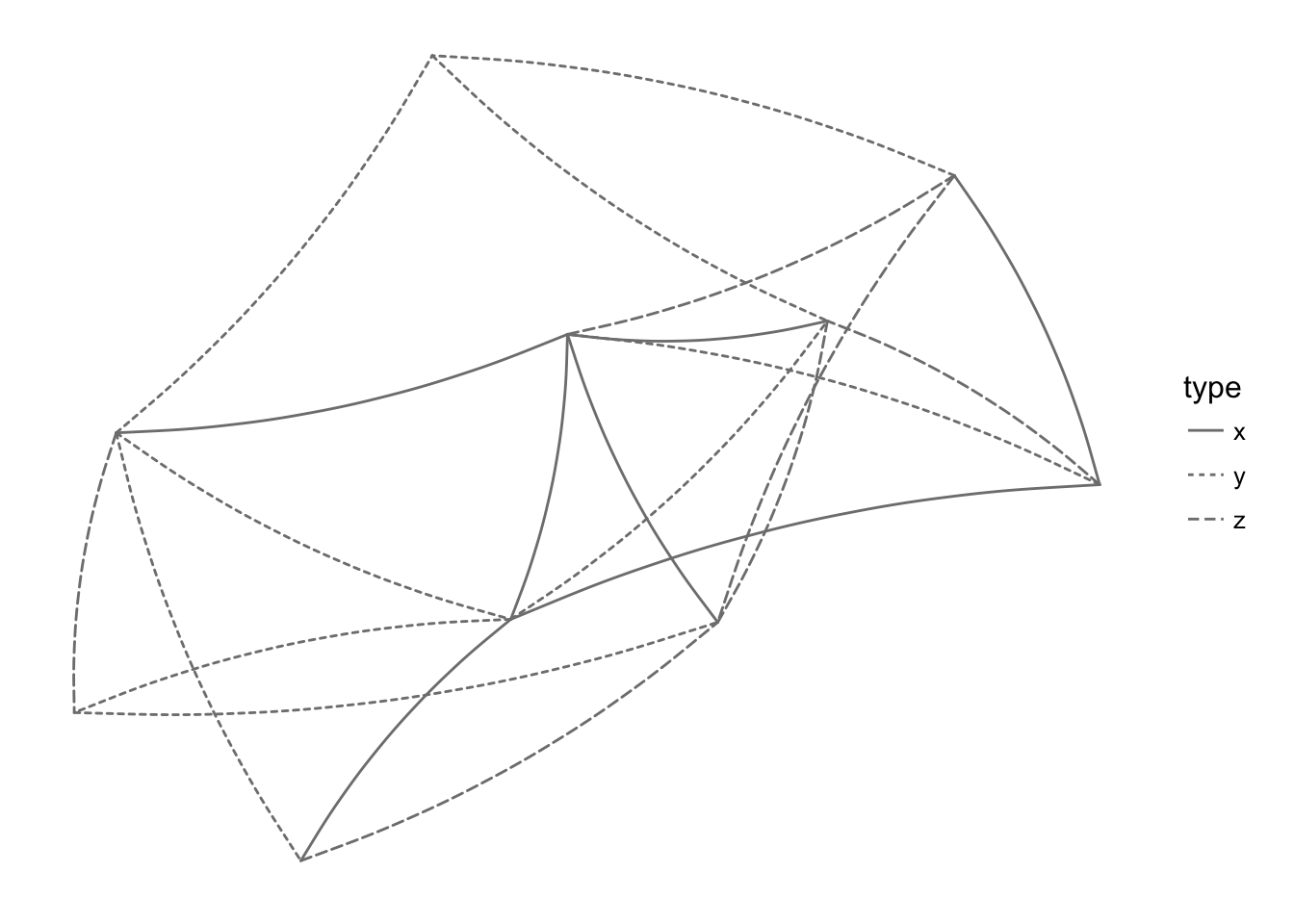The `ggnetwork` package provides a way to build network plots with `ggplot2`.

Install the stable version from CRAN:

``install.packages("ggnetwork")``

Or use `devtools` to install the latest version of the package from GitHub:

``devtools::install_github("briatte/ggnetwork")``

The package is meant to be used with `ggplot2` version 2.0.0 or above, so make sure that you update your version of `ggplot2` from CRAN before using `ggnetwork`:

``````install.packages("ggplot2")
library(ggplot2)``````

`ggnetwork` further requires the `network` and `sna` packages for network manipulation, and will also install the `ggrepel` package for repulsive label drawing.

The `ggnetwork` package is very much related to the development of `geom_net` by Samantha C. Tyner and Heike Hoffmann. It also shares some similarity to the `ggnet` and `ggnet2` functions, which are part of the `GGally` package by Barret Schloerke and others. Each of these projects are extensions to Hadley Wickham’s implementation of Leland Wilkinson’s “grammar of graphics” in ggplot2.

## Minimal example

Let’s define a small random graph to illustrate each component of `ggnetwork`:

``````library(network)
library(sna)
n <- network(rgraph(10, tprob = 0.2), directed = FALSE)``````

Let’s now add categorical and continuous attributes for both edges and vertices. We’ll start with nodes, adding a categorical vertex attribute called `"family"`, which is set to either `"a"`, `"b"` or `"c"`, and a continuous vertex attribute called `"importance"`, which is set to either 1, 2 or 3.

``````n %v% "family" <- sample(letters[1:3], 10, replace = TRUE)
n %v% "importance" <- sample(1:3, 10, replace = TRUE)``````

We now add a categorical edge attribute called `"type"`, which is set to either `"x"`, `"y"` or `"z"`, and a continuous vertex attribute called `"day"`, which is set to either 1, 2 or 3.

``````e <- network.edgecount(n)
set.edge.attribute(n, "type", sample(letters[24:26], e, replace = TRUE))
set.edge.attribute(n, "day", sample(1:3, e, replace = TRUE))``````

Last, note that `ggnetwork` contains a “blank” plot theme that will avoid plotting axes on the sides of the network. We will use that theme in most of the plots:

``theme_blank``
``````## function(base_size = 12, base_family = "", ...) {
##   ggplot2::theme_bw(base_size = base_size, base_family = base_family) +
##     ggplot2::theme(
##       axis.text = ggplot2::element_blank(),
##       axis.ticks = ggplot2::element_blank(),
##       axis.title = ggplot2::element_blank(),
##       legend.key = ggplot2::element_blank(),
##       panel.background = ggplot2::element_rect(fill = "white", colour = NA),
##       panel.border = ggplot2::element_blank(),
##       panel.grid = ggplot2::element_blank(),
##       ...
##     )
## }
## <environment: namespace:ggnetwork>``````

## Main building blocks

### `ggnetwork`

The `ggnetwork` package is organised around a ‘workhorse’ function of the same name, which will ‘flatten’ the network object to a data frame that contains the edge list of the network, along with the edge attributes and the vertex attributes of the sender nodes.

The network object referred to above might be an object of class `network`, or any data structure that can be coerced to it, such as an edge list, an adjacency matrix or an incidence matrix. If the `intergraph` package is installed, then objects of class `igraph` can also be used with the `ggnetwork` package.

The data frame returned by `ggnetwork` also contains the coordinates needed for node placement as columns `"x"`, `"y"`, `"xend"` and `"yend"`, which as a consequence are “reserved” names in the context of `ggnetwork`. If these names show up in the edge or the vertex attributes, the function will simply fail to work.

The default node placement algorithm used by `ggnetwork` to produce these coordinates is the Fruchterman-Reingold force-directed layout algorithm. All of the placement algorithms implemented in the `sna` package are available through `ggnetwork`, which also accepts additional layout parameters:

``````ggnetwork(n, layout = "fruchtermanreingold", cell.jitter = 0.75)
ggnetwork(n, layout = "target", niter = 100)``````

The `layout` argument will also accept user-submitted coordinates as a two-column matrix with as many rows as the number of nodes in the network.

The top of the data frame produced by `ggnetwork` contains self-loops to force every node to be included in the plot. This explains why the rows shown below have the same values in `"x"` and `"xend"` (and in `"y"` and `"yend"`), and only missing values in the columns corresponding to the edge attributes:

``head(ggnetwork(n))``
``````##           x          y family importance  na.x vertex.names      xend
## 1 0.1830104 0.42047953      c          2 FALSE            1 0.1830104
## 2 0.4690597 0.00000000      b          2 FALSE            2 0.4690597
## 3 1.0000000 0.25891124      c          3 FALSE            3 1.0000000
## 4 0.2814689 1.00000000      c          3 FALSE            4 0.2814689
## 5 0.0000000 0.50312529      a          2 FALSE            5 0.0000000
## 6 0.9306397 0.02183163      c          1 FALSE            6 0.9306397
##         yend day na.y type
## 1 0.42047953  NA   NA <NA>
## 2 0.00000000  NA   NA <NA>
## 3 0.25891124  NA   NA <NA>
## 4 1.00000000  NA   NA <NA>
## 5 0.50312529  NA   NA <NA>
## 6 0.02183163  NA   NA <NA>``````

The next rows of the data frame contain the actual edges:

``tail(ggnetwork(n))``
``````##            x         y family importance  na.x vertex.names      xend
## 18 0.8748765 0.2445744      a          2 FALSE            5 0.4203488
## 19 0.8748765 0.2445744      a          2 FALSE            5 0.4989169
## 20 1.0000000 0.7239847      c          2 FALSE           10 0.8748765
## 21 1.0000000 0.7239847      c          2 FALSE           10 0.7160749
## 22 1.0000000 0.7239847      c          2 FALSE           10 0.7091942
## 23 1.0000000 0.7239847      c          2 FALSE           10 0.4939615
##         yend day  na.y type
## 18 0.5530424   2 FALSE    z
## 19 0.0000000   2 FALSE    y
## 20 0.2445744   2 FALSE    x
## 21 0.6762465   3 FALSE    y
## 22 0.4029760   1 FALSE    z
## 23 0.8738652   1 FALSE    x``````

The data frame returned by `ggnetwork` has (N + E) rows, where N is the number of nodes of the network, and E its number of edges. This data format is very likely to include duplicate information about the nodes, which is unavoidable.

Note that `ggnetwork` does not include any safety mechanism against duplicate column names. As a consequence, if there is both a vertex attribute called `"na"` and an edge attribute called `"na"`, as in the example above, then the vertex attribute will be renamed `"na.x"` and the edge attribute will be renamed `"na.y"`.

### `fortify.network` and `fortify.igraph`

The ‘flattening’ process described above is implemented by `ggnetwork` as `fortify` methods that are recognised by `ggplot2`. As a result, `ggplot2` will understand the following syntax as long as `n` is an object of class `network` or of class `igraph`:

``ggplot(n)``

However, if the object `n` is a matrix or an edge list to be coerced to a network object, you are required to use the `ggnetwork` function to pass the object to `ggplot2`:

``ggplot(ggnetwork(n))``

### `geom_edges`

Let’s now draw the network edges using `geom_edges`, which is just a lightly hacked version of `geom_segment`. In the example below, we map the `type` edge attribute to the linetype of the network edges:

``````ggplot(n, aes(x = x, y = y, xend = xend, yend = yend)) +
geom_edges(aes(linetype = type), color = "grey50") +
theme_blank()``````The other aesthetics that we mapped are the basic coordinates of the network plot. These might also be set as part of the call to `geom_segment`, but setting them at the root of the plot avoids having to repeat them in additional geoms.

Note that `geom_edges` can also produce curved edges by setting its `curvature` argument to any value above `0` (the default):

``````ggplot(n, aes(x = x, y = y, xend = xend, yend = yend)) +
geom_edges(aes(linetype = type), color = "grey50", curvature = 0.1) +
theme_blank()``````### `geom_nodes`

Let’s now draw the nodes using `geom_nodes`, which is just a lightly hacked version of `geom_point`. In the example below, we map the `family` vertex attribute to the color of the nodes, and make the size of these nodes proportional to the `importance` vertx attribute:

``````ggplot(n, aes(x = x, y = y, xend = xend, yend = yend)) +
geom_edges(aes(linetype = type), color = "grey50") +
geom_nodes(aes(color = family, size = importance)) +
theme_blank()``````